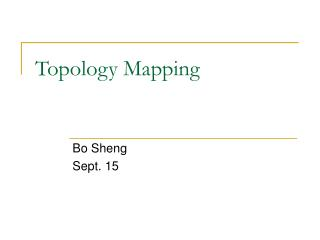DownloadDownload PresentationTopology Mapping

# Topology Mapping

Download Presentation## Topology Mapping

- - - - - - - - - - - - - - - - - - - - - - - - - - - E N D - - - - - - - - - - - - - - - - - - - - - - - - - - -
##### Presentation Transcript

1. Topology Mapping Bo Sheng Sept. 15

2. Outline • Overview • Solutions • LTM • ACE • Problems and discussion • Conclusion

3. Introduction • Topology mapping • Mismatch between overlay and physical infrastructure • Topology optimization

4. Introduction • Traffic problem • Facts • 95% of any pairs of Gnutella nodes are within 7 hops • 50,000 nodes generate 1G/second, 330T/month • Reasons • Blind flooding • Cycles, merge of multiple paths, neighbors exchange • Topology problem • Multiple times over a physical link

5. Introduction • Perfect match S S Network infrastructure Overlay network

6. Introduction • Mismatch N3 N1 4 5 2 3 S S 2 5 4 N2 Network infrastructure Overlay network

7. Topology Mismatch • Problems • Randomly choosing neighbors • Logically close, but physically far away S P N1 N2

8. Topology Mismatch • Problems • Unnecessary traffic • Inefficient utilization of bandwidth • Only 2%~5% Gnutella connections link nodes within a single AS (autonomous system) • More than 40% Gnutella nodes are located within top 10 AS • Delayed response • Do we need long-distance neighbors?

9. Topology Mismatch • Solutions to traffic problem • Selective flooding • Topology optimization • Avoid cycles • Mapping For each message, how many times it is delivered over a single physical link?

10. Performance Metrics • Traffic cost • Search scope • Response time • Overhead

11. Approaches • Location-aware Topology Matching (LTM), INFOCOM 2004 • Adaptive Connection Establishment (ACE), ICDCS 2004

12. LTM • Three main operations • TTL-2-detector flooding • Message format • Short Source IP& timestamp • Long Source IP& timestamp, TTL1 IP& timestamp • d(i,S,v) • Link cost IP(S),T(S) S N1 N2 IP(S),T(S) IP(N1),T(N1) d(i,S,1) d(i,S,0)

13. LTM • Three main operations • Low productive connection cutting • Case1: P receives d(i,S,1) and d(i,S,0) S N P will-cut list

14. LTM • Three main operations • Low productive connection cutting • Case2: P receives multiple d(i,S,0) S N1 N2 P

15. LTM • Three main operations • Low productive connection cutting • Case3: P receives one d(i,S,1) and multiple d(i,S,0) S N1 N2 P cut list

16. LTM • Three main operations • Source peer probing S N1 P

17. LTM Step2.case2 S S Step3 N1 N1 N2 P P

18. LTM Step2.case3 Step2.case2 S S N1 N1 N2 N2 P P Step2.case3 Step2.case1

19. LTM Step3 S S Step2.case1 N1 N1 P P

20. LTM • States Case2 Case1 Case3 Step3

21. LTM • Performance • Traffic • Search scope • Overhead

22. ACE • Step1: • Probe link costs with neighbors • Build neighbor cost table • Exchange neighbors cost table with neighbors

23. ACE • Step2: • Create a minimum spanning tree among each peer and its neighbors E E 14 14 4 4 15 G G S S 6 6 20 F F

24. ACE • Step3: • Replace neighbors Case1: SH<SG E Case2: GH>SH>SG 14 4 Case3: SH>SG,SH>GH G S 6 H F

25. ACE • Depth of optimization (h-neighbor closure) A 15 10 D 20 B 8 12 14 C E 7 A->B=10 A->D=15 E->C=7 E->D=14 B->E=8 D->E=14 Total:68

26. ACE • 2-neighbor closure A A 15 10 D D 20 B B 8 12 14 C E C E 7 A->B=10 B->E=8 E->C=7 E->D=14 Total:39

27. Discussion • Measurement • Link cost is not accurate • Link cutting and cycles • Heuristic to theoretical support • f (Pn,Tn)=?

28. Conclusion • Importance • Effectiveness vs. cost • Future work